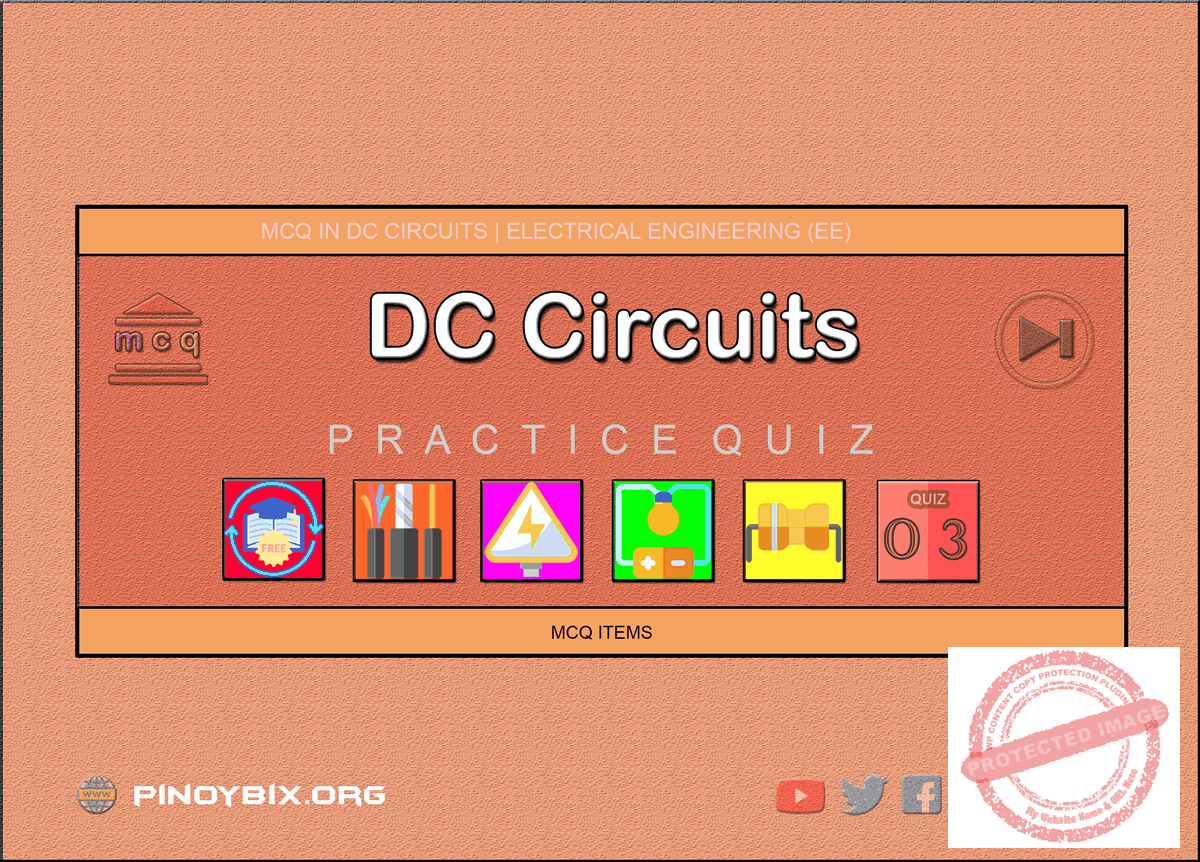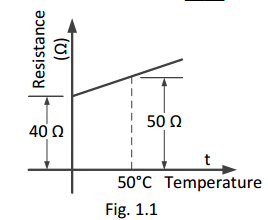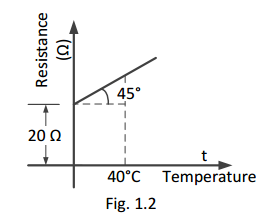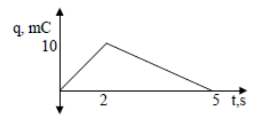# MCQ in DC Circuits Part 3 | REE Board Exam

(Last Updated On: November 13, 2020)This is the Multiple Choice Questions Part 3 of the Series in DC Circuits as one of the Electrical Engineering topic. In Preparation for the REE Board Exam make sure to expose yourself and familiarize in each and every questions compiled here taken from various sources including but not limited to past Board Exam Questions in Electrical Engineering field, Electrical Engineering Books, Journals and other Electrical Engineering References.

DC CIRCUITS: BASIC PRINCIPLES

#### Continue Practice Exam Test Questions Part 3 of the Series

Choose the letter of the best answer in each questions.

101.  A  certain  wire  20  ft  long  and 100  circular  mil area has a resistance of 1.6 Ω. What is its resistivity?

A.  10.3 ohm-CM/ft

B.  2.2 ohm-CM/ft

C.  8 ohm-CM/ft

D.  15.2 ohm-CM/ft

Solution:

102.  How many circular mils does a round copper rod of 0.25 inch diameter have?

A.  196,000

B.  62,500

C.  1,963,500

D.  49,000

Solution:

103.  A substance whose molecules consist of the same kind of atoms is called ______.

A.  mixture

B.  element

C.  compound

D.  none of these

Solution:

104.  The diameter of the atom is about ______.

A.  10-10 m

B.  10-8 m

C.  10-2 m

D.  10-15 m

Solution:

105.  The  number  of  compounds  available  in  nature  is ______.

A.  105

B.  300

C.  1000

D.  unlimited

Solution:

106.  The  mass  of  a  proton  is  ____  the  mass  of  an electron.

A.  equal to

B.  less than

D.  200 times

Solution:

107.  The  maximum  number  of  electrons  that  can  be accommodated in the last orbit is ____.

A.  4

B.  8

C.  18

D.  2

Solution:

108.  The electrons in the last orbit of an atom are called _____ electrons.

A.  free

B.  bound

C.  valence

D.  thermionic

Solution:

109.  If  the  number  of  valence  electrons  of  an  atom  is less than 4, the substance is usually ____.

A.  a conductor

B.  an insulator

C.  a semiconductor

D.  none of these

Solution:

110.  If  the  number  of  valence  electrons  of  an  atom  is more than 4, the substance is usually ____.

A.  a semiconductor

B.  a conductor

C.  an insulator

D.  none of these

Solution:

111.  If  the  number  of  valence  electrons  of  an  atom  is exactly 4, the substance is usually ____.

A.  a semiconductor

B.  an insulator

C.  a conductor

D.  a semiconductor

Solution:

112.  The number of valence electrons of an atom is less than 4. The substance will be probably ____.

A.  a metal

B.  a non-metal

C.  an insulator

D.  a semiconductor

Solution:

113.  One  coulomb  of  charge  is  equal  to  the  charge  on ____ electrons.

A.  628 x 1016

C.  62.8 x 1016

B.  6.28 x 1016

D.  0.628 x 1016

Solution:

114.  One  cc of copper has about _____ free electrons at room temperature.

A.  200

B.  20 x 1010

C.  8.5 x 1022

D.  3 x 105

Solution:

115.  Electric current in a wire is the flow of ______.

A.  free electrons

B.  bound electrons

C.  valence electrons

D.  atoms

Solution:

116.  EMF in a circuit is ______.

A.  cause current to flow

B.  maintains potential difference

C.  increases the circuit resistance

D.  none of these

Solution:

117.  EMF has the unit of _____.

A.  power

B.  energy

C.  charge

D.  none of these

Solution:

118.  Potential difference has the unit of _____.

A.  charge

B.  power

C.  energy

D.  none of these

Solution:

119.  The  resistance  of  a  material  is  ____  its  area  of cross-section.

A.  directly proportional to

B.  inversely proportional to

C.  independent of

D.  none of these

Solution:

120.  If the length and area of  cross-section of a wire are doubled up, then its resistance ______.

A.  becomes four times

B.  remains unchanged

C.  becomes sixteen times

D.  none of these

Solution:

121.  A  length  of  wire  has  a  resistance  of  6  ohms.  The resistance  of  a  wire  of  the  same  material  three times as long and twice the cross-sectional area will be ____.

A.  36 ohms

B.  12 ohms

C.  9 ohms

D.  1 ohm

Solution:

122.  The SI unit of specific resistance is ______.

A.  mho

B.  ohm-m

C.  ohm-m2

D.  ohm-cm

Solution:

123.  The  specific  resistance  of  a  conductor  ______  with rise in temperature.

A.  increases

B.  decreases

C.  remains unchanged

D.  none of these

Solution:

124.  The SI unit of conductivity is ______.

A.  ohm-m

B.  ohm/m

C.   mho-m

D.  mho/m

Solution:

125.  The SI unit of conductance is ______.

A.  mho

B.  ohm

C.  ohm-m

D.  ohm-cm

Solution:

126.  The resistance of a material 2 m long and 2 m2 in cross-sectional  area  is  1.6  x  10-8 Ω.  Its  specific resistance will be ______.

A.  3.2 x 10-8 ohm-m

B.  6.4 x 10-8 ohm-m

C.  1.6 x 10-8 ohm-m

D.  0.16 x 10-8 ohm-m

Solution:

127.  Conductors  have  ______  temperature  coefficient  of resistance.

A.  positive

B.  negative

C.  zero

D.  none of these

Solution:

128.  Semiconductors have ______ temperature coefficient of resistance.

A.  negative

B.  positive

C.  zero

D.  none of these

Solution:

129.  The  value  of  α  (i.e.  temperature  coefficient  of resistance) depends upon

A.  length of the material

B.  cross-sectional area of the material

C.  volume of the material

D.  nature of the material and temperature

Solution:

130.  The  temperature  coefficient  of  resistance  of  a conductor ______ with rise in temperature.

A.  increases

B.  decreases

C.  remains unchanged

D.  none of these

Solution:

131.  Insulators  have  ______  temperature  coefficient  of resistance.

A.  zero

B.  positive

C.  negative

D.  none of these

Solution:

132.  Eureka  has  ______  temperature  coefficient  of resistance.

A.  positive

B.  negative

C.  almost zero

D.  none of these

Solution:

133.  Fig. 1.1 shows the temperature/resistance graph of a conductor. The value of α0 is ______.A.  0.005/°C

B.  0.004/°C

C.  0.1/°C

D.  0.4/°C

Solution:

134.  Referring  to  Fig.  1.1,  the  value  of  the  α50  will  be ______.A.  0.005/°C

B.  0.004/°C

C.  0.1/°C

D.  0.4/°C

Solution:

135.  Referring to Fig. 1.2, the value of α0 is ______.A.  1/30 per °C

B.  1/40 per °C

C.  1/1200 per °C

D.  none of these

Solution:

136.  Referring to Fig. 1.2, the value of R40 will be _____.A.  70 ohms

B.  40 ohms

C.  35 ohms

D.  50 ohms

Solution:

137.  Referring to Fig. 1.2, the value of α40 will be _____.A.  1/30 per °C

B.  1/70 per °C

C.  1/50 per °C

D.  1/1200 per °C

Solution:

138.  The value of α0  of a conductor is 1/236 per °C. The value of α18 will be _____.

A.  1/218 per °C

B.  1/272 per °C

C.  1/254 per °C

D.  none of these

Solution:

139.  The value of α50 of a conductor is 1/230 per °C. The value of α0 will be ____.

A.  1/180 per °C

B.  1/280 per °C

C.  1/250 per °C

D.  none of these

Solution:

140.  A good electric conductor is one that

A.  has low conductance

B.  is always made of copper wire

C.  produces a minimum voltage drop

D.  has few free electrons

Solution:

141.  Two  wires  A  and  B  have  the  same  cross-section and are made of the same material, RA = 600 Ω and RB = 100 Ω. The number of times A is longer than B is

A.  6

B.  2

C.  4

D.  5

Solution:

142.  A coil has a resistance of 100 Ω  at 90°C. At 100°C, its resistance is 101  Ω. The temperature coefficient of wire at 90°C is

A.  0.01

B.  0.1

C.  0.0001

D.  0.001

Solution:

143.  Which  of  the  following  material  has  nearly  zero temperature-coefficient of resistance?

A.  carbon

B.  porcelain

C.  copper

D.  manganin

Solution:

144.  Which  of  the  following  material  has  a  negative temperature coefficient of resistance?

A.  brass

B.  copper

C.  aluminum

D.  carbon

Solution:

145.  A cylindrical wire 1 m in length, has a resistance of 100  Ω.  What  would  be  the  resistance  of  a  wire made  from  the  same  material  both  the  length  and the cross-sectional area are doubled?

A.  200

B.  400

C.  100

D.  50

Solution:

146.  Carbon  composition  resistors  are  most  popular because they

A.  cost the least

B.  are smaller

D.  do not produce electric noise

Solution:

147.  A unique feature of a wire-wound resistor is its

A.  lower power rating

B.  low cost

C.  high stability

D.  small size

Solution:

148.  A  coil  has  a  resistance  of  100  ohms  at  90°C.  At 100°C,  its  resistance  is  101  ohms.  What  is  the temperature coefficient of the wire at 90°C?

A.  0.01

B.  0.1

C.  0.0001

D.  0.001

Solution:

149.  What is the unit for charge (Q)?

B.  Joule

C.  Siemens

D.  Coulomb

Solution:

150.  The charge delivered by a constant voltage source is  shown.  Determine  the  current  supplied  by  the source at (a) t = 1 s (b) t = 3 s.A.  5 ma, -3.33 ma

B.  5 ma, 3.33 ma

C.  –3.33 ma, 5 ma

D.  3.33 ma, 5 ma

Solution:

#### Questions and Answers in DC Circuits

Following is the list of practice exam test questions in this brand new series:

MCQ in DC Circuits
PART 1: MCQ from Number 1 – 50                Answer key: included
PART 2: MCQ from Number 51 – 100            Answer key: included
PART 3: MCQ from Number 101 – 150           Answer key: included
PART 4: MCQ from Number 151 – 200           Answer key: included
PART 5: MCQ from Number 201 – 250           Answer key: included
PART 6: MCQ from Number 251 – 300           Answer key: included
PART 7: MCQ from Number 301 – 350           Answer key: included
PART 8: MCQ from Number 351 – 400            Answer key: included
PART 9: MCQ from Number 401 – 450            Answer key: included
PART 10: MCQ from Number 451 – 500          Answer key: included

PinoyBIX Engineering. © 2014-2020 All Rights Reserved | How to Donate? |